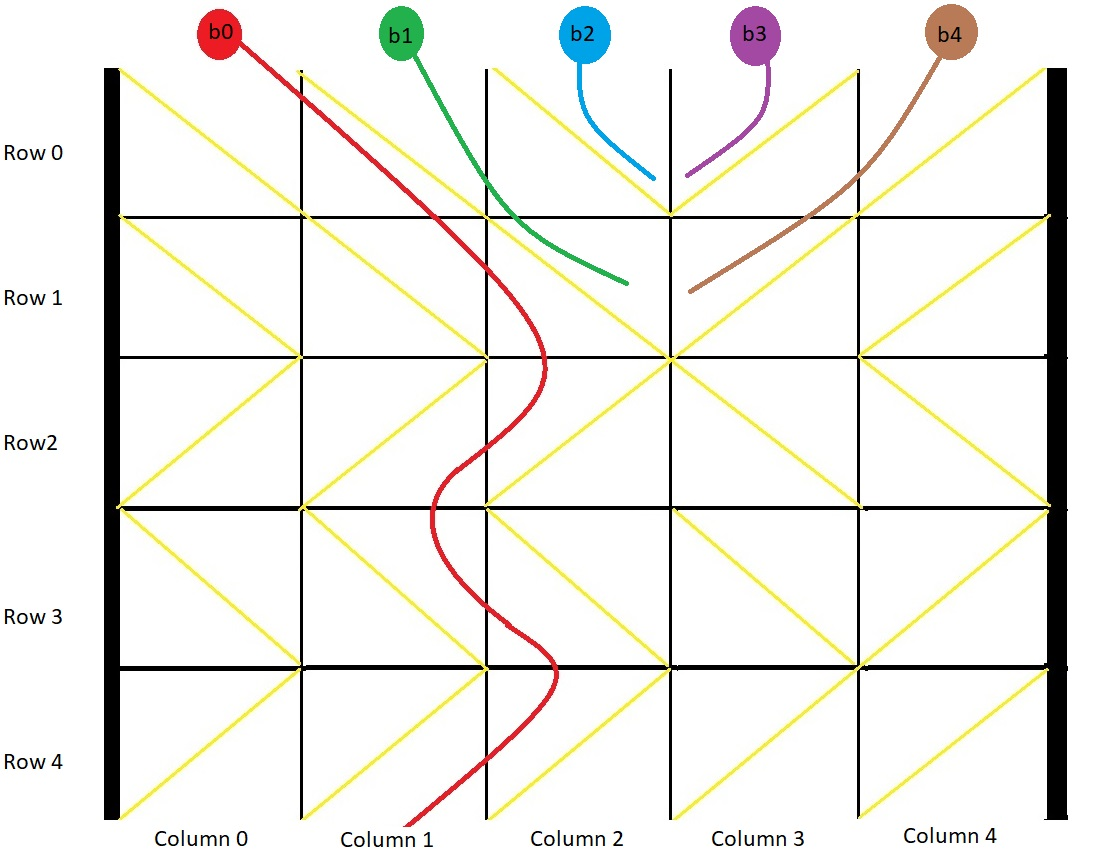# 1706. Where Will the Ball Fall

Medium
You have a 2-D `grid` of size `m x n` representing a box, and you have `n` balls. The box is open on the top and bottom sides.
Each cell in the box has a diagonal board spanning two corners of the cell that can redirect a ball to the right or to the left.
• A board that redirects the ball to the right spans the top-left corner to the bottom-right corner and is represented in the grid as `1`.
• A board that redirects the ball to the left spans the top-right corner to the bottom-left corner and is represented in the grid as `-1`.
We drop one ball at the top of each column of the box. Each ball can get stuck in the box or fall out of the bottom. A ball gets stuck if it hits a "V" shaped pattern between two boards or if a board redirects the ball into either wall of the box.
Return an array `answer` of size `n` where `answer[i]` is the column that the ball falls out of at the bottom after dropping the ball from the `ith` column at the top, or `-1` if the ball gets stuck in the box.
Example 1:Input: grid = [[1,1,1,-1,-1],[1,1,1,-1,-1],[-1,-1,-1,1,1],[1,1,1,1,-1],[-1,-1,-1,-1,-1]]
Output:
[1,-1,-1,-1,-1]
Explanation:
This example is shown in the photo.
Ball b0 is dropped at column 0 and falls out of the box at column 1.
Ball b1 is dropped at column 1 and will get stuck in the box between column 2 and 3 and row 1.
Ball b2 is dropped at column 2 and will get stuck on the box between column 2 and 3 and row 0.
Ball b3 is dropped at column 3 and will get stuck on the box between column 2 and 3 and row 0.
Ball b4 is dropped at column 4 and will get stuck on the box between column 2 and 3 and row 1.
Example 2:
Input: grid = [[-1]]
Output:
[-1]
Explanation:
The ball gets stuck against the left wall.
Example 3:
Input: grid = [[1,1,1,1,1,1],[-1,-1,-1,-1,-1,-1],[1,1,1,1,1,1],[-1,-1,-1,-1,-1,-1]]
Output:
[0,1,2,3,4,-1]
Constraints:
• `m == grid.length`
• `n == grid[i].length`
• `1 <= m, n <= 100`
• `grid[i][j]` is `1` or `-1`.

### 解題

Runtime: 26 ms, faster than 85.78%
Memory Usage: 6.6 MB, less than 90.69%
func findBall(grid [][]int) []int {
res := make([]int, 0)
for i:=0; i<len(grid); i++ {
res = append(res, dfs(0, i, grid))
}
return res
}
func dfs(x int, y int, grid [][]int) int {
if x == len(grid) { return y }
if grid[x][y] == 1 {
if y == len(grid) - 1 || grid[x][y+1] == -1 {
return -1
}
return dfs(x+1, y+1, grid)
} else {
if y == 0 || grid[x][y-1] == 1 {
return -1
}
return dfs(x+1, y-1, grid)
}
return 0
}